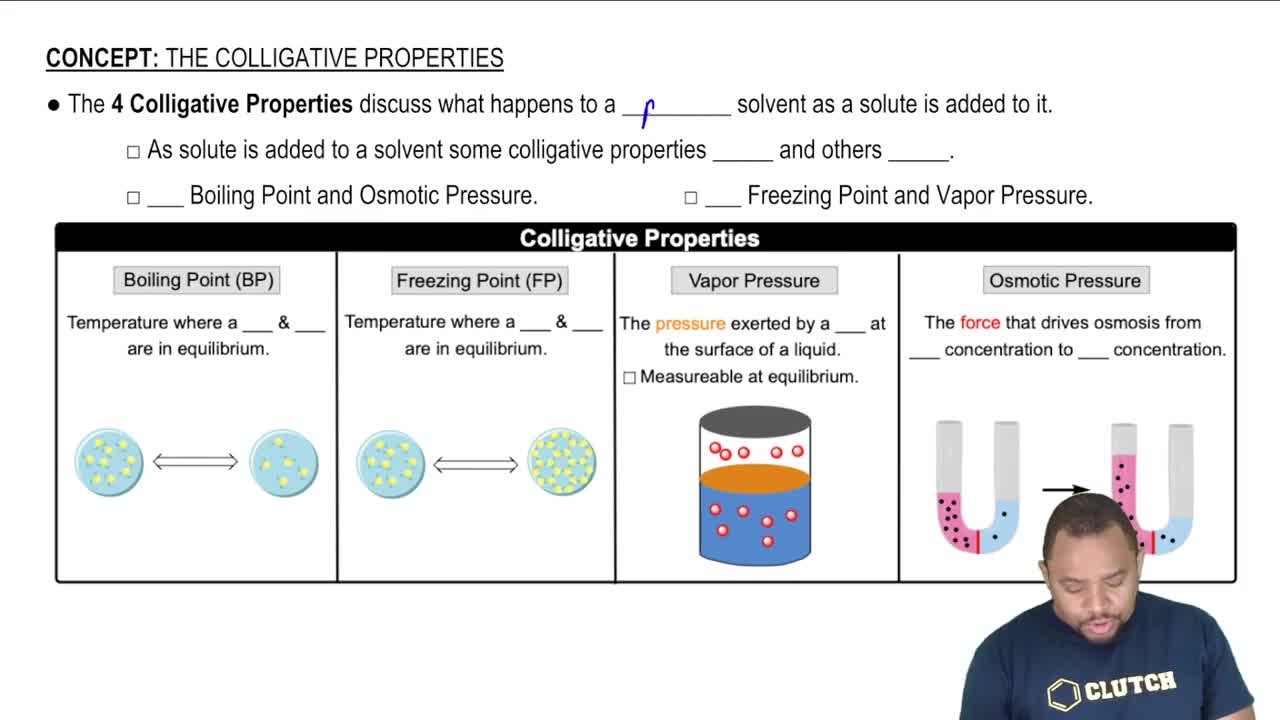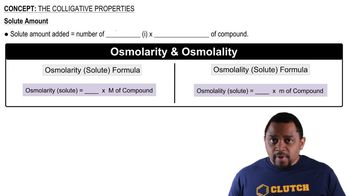Start typing, then use the up and down arrows to select an option from the list.# The Colligative Properties Example 3

Jules Bruno
170views
2
1
What is the Ionic morality of potassium ions in 1.1. A mobile solution. Potassium phosphate. Alright, So we want the ionic morality of potassium ions. Alright, So we're gonna say ionic morality is just osmolarity. So here it will equal the ions of potassium ion times the morality of the compound. If we break this up, it breaks up into three potassium ions, plus one phosphate ion. So how many potassium ions do we have? We have three, So that'd be three times now. The morality of the entire compound is 1.8 mol. So three times 1.18 Molo gives us the Ionic morality of potassium ions. So that will give us option E As our correct answer. So it'll be 3.54 model of potassium ions.02:4300:5002:5001:0501:2601:03